# Multiply Numbers by Drawing Lines

This is awesome:

Metacafe vodeo link found via haha.nu

I think this could be a cool educational tool to teach kids multiplication on large numbers. I’m guessing there is some mathematical theory that proves how it works.

[tags]lifehack, multiplication, cool, drawing[/tags]

This entry was posted in Uncategorized and tagged . Bookmark the permalink.

### 6 Responses to Multiply Numbers by Drawing Lines

1.ambush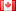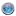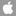says:

there is a mathematical theory and it is deceptively simple, you are essentially multiplying each individual digit out where the lines cross.

2.Matt`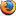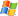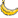says:

I figured it was probably something along those lines – you multiply the end, then the middle (which will be the sum of 2 multiplications) then the front

hmm… if the digit for one set of crossovers was over 10, you’d need to carry some over into the next column, otherwise it would make a 4 or 5 digit number

3.Luke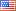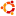says:

Yeah, that’s how if works, but why? I would love to see a mathematical proof of this.

4.Matt`says:

You do the same thing with the lines as you do with long multiplication – just layed out differently.

(From right to left on line diagram or on long multiplication columns)
First multiply the 2 2nd digits, thats the end digit of the result
Then multiply the 1st digit with the 2nd, and the 2nd with the 1st, and add the 2 resulting numbers, thats the 2nd digit of the result
Then multiply the 1st digit in each number and thats the 1st digit in the answer

The proof isnt so much mathematical as logical

Kinda similar to the “grid method” that we got taught once – instead of “234 x 123” you do 200×100, 200×20, 200×3, 30×100, 30×20, 30×3, 4×100, 4×20 and 4×3 then add up all the results, but its shown as a grid with “200”, “30” and “4” as the column headings and “100”, “20” and “3” as the row titles… if you see what I mean

5.Auto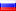says:

Yes such mathematics useful in the program when I Photoshop working on my head around so the figures fall

6.Luke Maciaksays:

I’m pretty sure Auto’s comment is a spam, but I approved it for the comedic value of Bablefish translation! LOL!